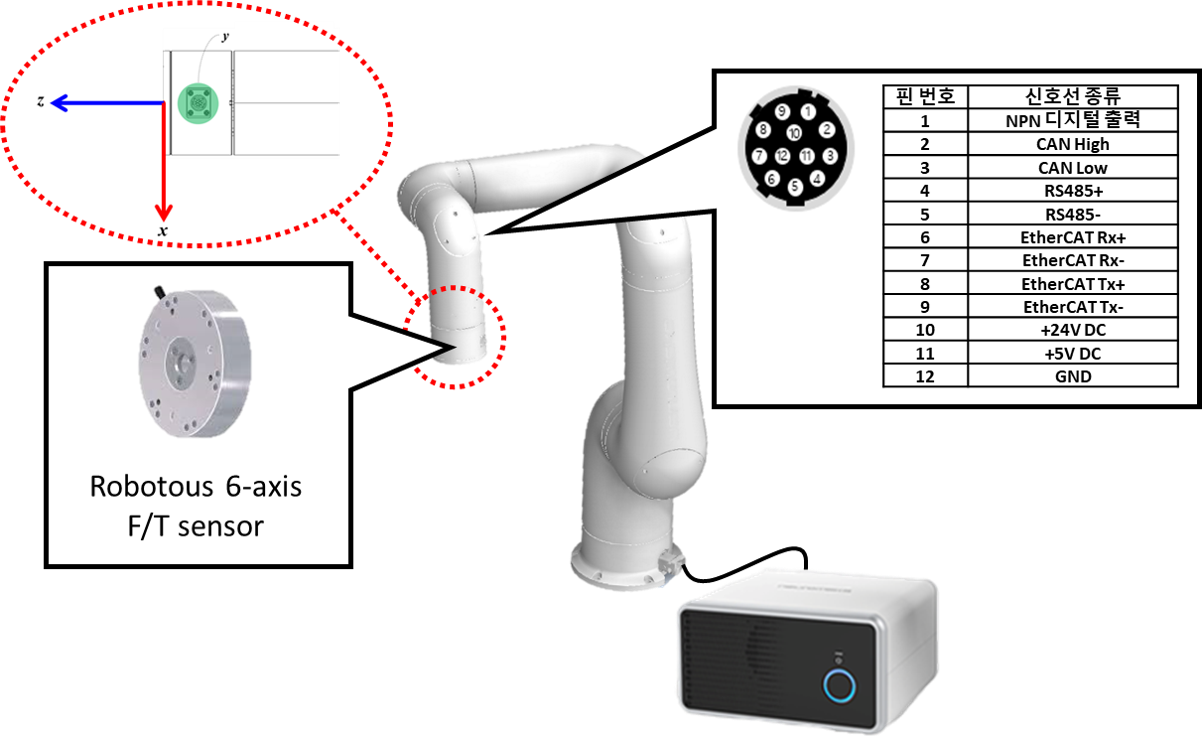# F/T-based Friction Compensation

## System Configuration

This section describes how to initialize the bias of the F/T sensor and transform it's coordinate by implementing the practical control example. To this end, a robot with F/T sensors installed at the end-effector (or end-tool) is considered throughout the example. Note that the F/T sensor is installed to align its x-axis with the x-axis of the end-effector.System configuration to implement F/T sensor-based control

This example investigates the followings:

• How to transform the coordinate of the F/T sensor.
• How to implement inertia reshaping control utilizing transformed F/T sensor data.

## Example Code

As a first step, generate the joint control component. Then, to handle shared memory, add the relevant headers and declare the ShmemManager-type pointer like follows (for more details on acquiring F/T sensor data, refer to F/T sensor and gripper):

 1 2 3 4 5 #include NRMKFramework::ShmemManager * indyShm = NULL; NRMKIndy::SharedData::ExtraIOData extioData; NRMKIndy::SharedData::RobotControlSharedData ctrData; 

Define the following variables and functions in the joint control component header.

  1 2 3 4 5 6 7 8 9 10 11 12 13 14 15 16 17 // typedef typename ROBOT::JointMat JointMat; // typedef NRMKFoundation::CompositeHTransformTaskPosition TaskPosition; // typedef NRMKFoundation::CompositeHTransformTaskVelocity TaskVelocity; // typedef Eigen::Matrix TaskVec; //!< Typedef of task vector typedef Eigen::Matrix TaskJac; //!< Typedef of task Jacobian matrix void readFTSensor(ROBOT & robot); void setBias(){_ftBias = _ftSensor;} TaskPosition _tposT; TaskVelocity _tvelT; LieGroup::Wrench _ftBias; LieGroup::Wrench _ftSensor; LieGroup::Rotation _ft_sensor_orientation; 

Then, in constructor and destructor, add the code that allocates and releases the memory of ShmemManager-type pointer variable indyShm.

 1 2 3 4 5 6 JointControlComponent::JointControlComponent() : AbstractController(USERNAME, EMAIL, SERIAL) , _robotNom(NULL) { indyShm = new NRMKFramework::ShmemManager(INDY_SHM_NAME, INDY_SHM_LEN); } 
 1 2 3 4 5 6 7 8 JointControlComponent::~JointControlComponent() { if (_robotNom != NULL) delete _robotNom; // TODO Auto-generated destructor stub if (indyShm != NULL) delete indyShm; } 

Set the relative coordinates between the F/T sensor and end-effector (or end-tool) coordinates using setTFTSensor() function. To help readers understand, two coordinate setting cases are given in the following code.

• Case 1: F/T sensor's x/y-axis is set in the opposite direction to the end-effector's x/y-axis while the z-axis of them is installed to be coincided each other.

• Case 2: F/T sensor is rotated by the amount of -5.0/12*$\pi$ radian in the z-axis direction, compared to the first case.

Note that, in this example, our case corresponds to the first case and the displacement is set to zero because the F/T sensor is directly attached to the end-effect.

  1 2 3 4 5 6 7 8 9 10 11 12 13 14 void JointControlComponent::initialize(ROBOT & robot, double delt) { //define nominal robot (this is not the consideration of this example) _robotNom = AbstractController::createRobotObj(); //example 1. _ft_sensor_orientation = LieGroup::Rotation(-1,0,0,0,-1,0,0,0,1); //example 2. //_ft_sensor_orientation = LieGroup::Rotation(2, -5.0/12.0*M_PI). //cascade(LieGroup::Rotation(-1,0,0,0,-1,0,0,0,1)); robot.setTFTSensor(ft_sensor_orientation, LieGroup::Displacement::Zero()); } 

Let assume that the position controller is well constructed and apply the inertia reshaping control for the direct teaching. The objective of inertia reshaping control is to reduce the inertia of the robot and attenuate the effect of friction. To implement this, copy the following code into the computeGravityTorq() function (for details on the position controller implementation, refer to Nonlinear Optimal Control Example).

• Define readFTsensor function to get F/T sensor data and transform its coordinate.
• Calculate the gravity force with idyn_gravity function to compensate it.
• Calculate the forward kinematics.
• Get the Jacobian matrix to transform the force acting on the end-effect to the force applied to the joint.
• This example aims to reduce the effect of inertia and friction. Therefore, the control input (u) is set to zero.
  1 2 3 4 5 6 7 8 9 10 11 12 13 14 15 16 17 18 19 20 21 22 23 24 25 26 27 28 29 30 31 32 33 34 35 36 37 int IntertiaReshapingwFTsensor::computeGravityTorq(ROBOT & robot, LieGroup::Vector3D const & gravDir, JointVec & torque) { if (indyShm == NULL) { printf("Shared memory is missed\n"); } //read F/T sensor readFTSensor(robot); //calculate torque control input to compensate for the effect of friction JointVec u, tauGrav, tauExt, K_ir; TaskJacobian J, Jdot; //calculate the gravity robot.idyn_gravity(LieGroup::Vector3D(0, 0, -GRAV_ACC)); tauGrav = robot.tau(); //calculate forward kinematics and jacobian robot.computeFK(_tposT, _tvelT); robot.computeJacobian(_tposT, _tvelT, J, Jdot); //calculate external torque from measured external force tauExt = J.transpose()*_ftSensor; //define new control input: in this example, this is set to zero u.setZero(); //set inertia and friction reshaping gains K_ir << 5.0, 5.0, 3.0, 3.0, 3.0, 3.0; //update final control input torque = tauGrav - tauExt + K_ir.cwiseProduct(u + tauExt); return true; } 

readFTSensor is defined to get the F/T sensor data from a shared memory and transform the coordinate of acquired data to the global one, given like follows:

• Use getControlData function to get F/T sensor data and calculate its bias.
• Transform the F/T sensor values (represented in the F/T sensor coordinate) to the values represented in the target (end-effector) coordinate.
• Represent transformed F/T sensor values for the global coordinate.
  1 2 3 4 5 6 7 8 9 10 11 12 13 14 15 16 17 18 19 20 21 22 23 24 25 26 27 void JointControlComponent::readFTSensor(ROBOT & robot) { NRMKIndy::SharedData::getExtraIOData(*indyShm, extioData); NRMKIndy::SharedData::getControlData(*indyShm, ctrData); //reflect the biased force value LieGroup::Wrench _ftSensorRaw; for (int i=0; i<6; i++) _ftSensorRaw[i] = ctrData.Fext[i]; _ftSensorRaw -= _ftBias; //transform the coordinate // * Tft: transformation from reference coordinate to f/t sensor // * Ttarget: transformation from reference coordinate to end effector LieGroup::HTransform T_ft_target = robot.Ttarget().icascade(robot.Tft()); LieGroup::Wrench _ftSensorTemp = T_ft_target.itransform(_ftSensorRaw); TaskPosition _tartget_pos; TaskVelocity _tartget_vel; robot.computeFK(_tartget_pos, _tartget_vel); LieGroup::Wrench _ftSensorTransformed = LieGroup::Wrench(_tartget_pos.R()*_ftSensorTemp.f(), _ftSensorTemp.n()); for (int i=0; i<3; i++) _ftSensor[i] = _ftSensorTransformed[i+3]; for (int i=0; i<3; i++) _ftSensor[i+3] = _ftSensorTransformed[i]; } 

In the case that the sensitive force control is required or the repeatability of the F/T sensor is not guaranteed, add the following code to update the bias value when restarting the task:

 1 2 3 4 5 6 7 void JointControlComponent::reset() { NRMKIndy::SharedData::getExtraIOData(*indyShm, extioData); NRMKIndy::SharedData::getControlData(*indyShm, ctrData); for (int i=0; i<6; i++) _ftSensor[i] = ctrData.Fext[i]; setBias(); }